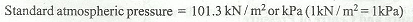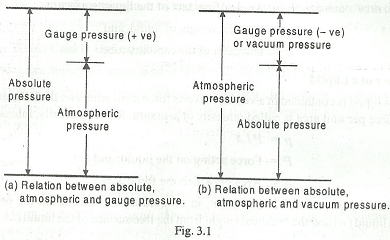### Atmospheric Pressure, Gauge Pressure and Absolute Pressure

The atmospheric air exerts a normal pressure upon all surfaces with which it is in contact and it is known as atmospheric pressure. It is also known as barometric pressure. The atmospheric pressure at sea level (above absolute zero) is called standard atmospheric pressure and its value is given as follows := 10.3 m of water
= 760 mm of Hg

The pressure measured with the help of a pressure gauge is known as guage pressure, in which atmospheric pressure is taken as datum. All the pressure gauges record the difference between the actual pressure and the atmospheric pressure. The actual pressure is known as absolute pressure.

Mathematically

Absolute pressure = Atmospheric pressure + Gauge pressure (Positive)

This relation is used for pressures above atmospheric, i.e. for positive gauge pressure, as shown in Fig. 3.1 (a), For pressures below atmospheric, the gauge pressure will be negative. This negative gauge pressure is known as vacuum pressure. Therefore

Absolute pressure = Atmospheric pressure - Negative gauge pressure or vacuum pressure

This relation is shown in Fig 3.1 (b).